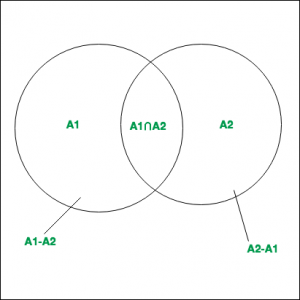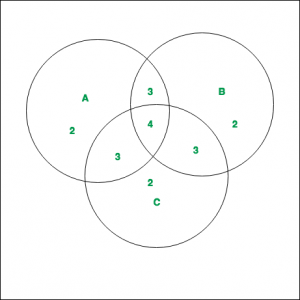# Inclusion-Exclusion and its various Applications

In the field of Combinatorics, it is a counting method used to compute the cardinality of the union set. According to basic Inclusion-Exclusion principle:

• For 2 finite setsand, which are subsets of Universal set, thenandare disjoint sets.Hence it can be said that,.

• Similarily for 3 finite sets,and,## Principle :

Inclusion-Exclusion principle says that for any number of finite sets, Union of the sets is given by = Sum of sizes of all single sets – Sum of all 2-set intersections + Sum of all the 3-set intersections – Sum of all 4-set intersections .. +Sum of all the i-set intersections.

In general it can be said that,Properties :

1. Computes the total number of elements that satisfy at least one of several properties.
2. It prevents the problem of double counting.

Example 1:
As shown in the diagram, 3 finite sets A, B and C with their corresponding values are given. Compute.Solution :
The values of the corresponding regions, as can be noted from the diagram are –By applying Inclusion-Exclusion principle,#### Applications :

• Derangements
To determine the number of derangements( or permutations) of n objects such that no object is in its original position (like Hat-check problem).
As an example we can consider the derangements of the number in the following cases:
For i = 1, the total number of derangements is 0.
For i = 2, the total number of derangements is 1. This is.
For i = 3, the total number of derangements is 2. These areand.
• Applying the Inclusion-Exclusion principle to i general eventsand rearranging we get the formula,My Personal Notes arrow_drop_upCheck out this Author's contributed articles.

If you like GeeksforGeeks and would like to contribute, you can also write an article using contribute.geeksforgeeks.org or mail your article to contribute@geeksforgeeks.org. See your article appearing on the GeeksforGeeks main page and help other Geeks.

Please Improve this article if you find anything incorrect by clicking on the "Improve Article" button below.